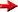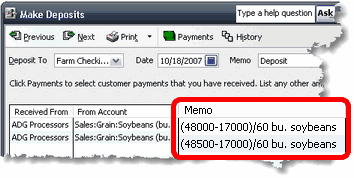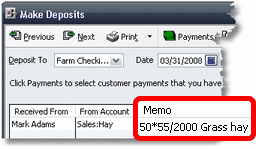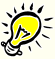Besides letting you enter quantities in the Memo or Description field of QuickBooks transactions, ManagePLUS lets you enter quantities there as mathematical formulas, which gives you several benefits:

 ü You can enter transactions without needing a calculator or using QuickBooks’ internal calculator feature to do quantity calculations.
 ü With the quantity represented as a formula instead of a single number you’ll more easily notice calculation errors, because all details of the calculation remain visible as part of the transaction—unlike when you’ve figured something on a calculator.
 ü Because all formulas details remain a part of the transaction, you can easily edit the formula later if you notice that it’s incorrect.
 ü Having the quantity stored as a formula in the transaction can be valuable information when you’re trying to recall transaction details later.Entering quantities as formulas works with ManagePLUS because the formulas you enter in the Memo/Description field will be processed later when you refresh transactions from QuickBooks into ManagePLUS. But you cannot store formulas in the Quantity column of a QuickBooks form…QuickBooks doesn’t support that.

ManagePLUS Mathematical Symbols

If you are familiar with spreadsheets, you will notice that quantity formulas in ManagePLUS are much like spreadsheet formulas, except ManagePLUS formulas are more limited. You can use the simple mathematical operators shown below, but you can can string together several factors of a calculation and use parentheses to control the calculation order.

ManagePLUS Mathematical Symbols
(listed in order of mathematical precedence)

( )

parentheses
(to control calculation order)

^

exponentiation

* /

multiplication, division

+ -

Entering quantities as mathematical formulas

 · Example #1:  Calculating a quantity in bushels.
 Here’s a Deposit showing quantities entered as formulas. In this example, quantities for the Sales:Grain:Soybeans (bu.) account need to be entered in bushels. (In fact, the abbreviation “bu.” has been made part of the account name to serve as reminder of the appropriate unit of measure for this account.)The formula in the first detail line is (48000/17000)/60. Here’s what it means:
 48000 The actual gross weight of the truckload of soybeans
 17000 The tare or “empty” weight of the truck
 60 The standard weight, in pounds, of a bushel of soybeans.
 48000-17000 = 31000 net pounds of soybeans.
 / 60 (divide) = 516.67 bushels, the quantity ManagePLUS will extract from this transaction.
 · Example #2:  Estimating tons from a count, and an average weight per item.
 A hay producer normally sells hay priced per ton, by the semi-truck load. Each load is weighed, so he keeps track of hay sales by the ton. But he also makes a few sales to individual horse hay customers, in small quantities. These small loads are usually sold “by the bale”. That is, the loads aren’t weighed, and the price is determined by the number of bales sold, times a price per bale.
 To have good quantity information at year’s end, he needs to record all hay sales in the same unit of measure—tons, in this case. How can he do that for hay sold “by the bale”? With ManagePLUS, the simple solution is to enter the quantity as a formula which estimates tons based on the number of bales sold and the estimated average weight per bale:Here’s what the formula means:
 50 The number of bales sold.
 55 The estimated average weight per bale, in pounds.
 2000 The number of pounds in a ton.
 50 * 55 (multiply) = 2750 pounds of hay sold.
 / 2000 (divide) = 1.375 tons, the quantity ManagePLUS will extract from this transaction.Entering the quantity as a formula automatically preserves transaction detail. In this case, when looking back at the transaction weeks or months later we could immediately know that it was for a sale of 50 bales of hay, averaging about 55 pounds each.